# ANALYSIS OF CLASSIFIED DATA

## ANALYSIS OF CLASSIFIED DATA

The purpose of this section is to:

1. Discuss the classified attribute analysis and classified variable analysis approaches to analyzing classified responses.

2. Present examples of how these techniques are used.

### CLASSIFIED RESPONSES

Some experimental responses cannot be measured on a continuous scale although they can be divided into sequential classes. Examples include appearance and performance ratings. In these situations, three to five rating classes are generally the optimum number because this number allows major differences in the responses to be identified and yet does not require the rater to identify differences that are too subtle. Two related techniques are used to analyze classified responses:

1. Classified attribute analysis is used when the total number of items rated is the same for every test matrix setup.

2. Classified variable analysis is used when the total number of items rated is not the same for every test matrix setup.

Three to five responses at each experimental setup are recommended to give a good evaluation of the class distribution of responses at that setup. As with continuous measurements, more responses at each setup allow smaller differences to be identified.

### CLASSIFIED ATTRIBUTE ANALYSIS

This technique converts the observed frequency in each class into a cumulative frequency for the classes. As an example, if there are three classes, the observed and cumulative frequencies might be as shown in Table 9.33.

Table 9.33: Observed Versus Cumulative Frequency

Observed Frequency

Cumulative Frequency

Class I

2

2

Class II

1

3

Class III

1

4

It is assumed that the user will use a computer program to analyze the classified data. The specific input format will depend on the computer program used. The mathematical derivations and philosophies of this approach will not be presented here. For more information see Volume V of this series as well as Taguchi (1987) and Wu and Moore (1985).Three grades are used to evaluate paint appearance of a product. They are "Bad," "OK," and "Good." Seven factors (A through G), each at two levels, are evaluated to determine the combination of factor levels that optimizes paint appearance. Five products are evaluated at each testing situation in an L8 orthogonal array. Test results are shown in Table 9.34.

Table 9.34: Attribute Test Setup and Results

A

B

C

D

E

F

C

OK

Good

1

1

1

1

1

1

1

2

3

1

1

1

2

2

2

2

3

2

1

2

2

1

1

2

2

4

1

1

2

2

2

2

1

1

2

3

2

1

2

1

2

1

2

4

1

2

1

2

2

1

2

1

1

3

1

2

2

1

1

2

2

1

3

2

2

2

1

2

1

1

2

1

4

The ANOVA analysis for this set of data is shown in Table 9.35. Note that the degrees of freedom are calculated differently from the non-classified situation. The df of each source is:

• (the number of levels of that factor - 1) * (the number of classes - 1)

Table 9.35: ANOVA Table (for Cumulative Frequency)

Source

df

SS

MS

F Ratio

S'

%

A

2

11.668

5.834

7.820

10.179

12.72

B

2

6.678

3.39

4.476

5.186

6.48

C

2*

0.125

0.063

D

2*

3.668

1.834

E

2*

2.259

1.130

F

2

7.935

3.986

5.319

6.443

8.05

G

2*

2.259

1.130

Error

64

45.409

0.710

(pooled error)

72

53.720

0.746

58.196

72.75

Total

78

80.000

1.026

In this example, the number of levels of each factor is two and the number of classes is three. For each factor,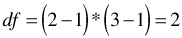The total df = (the total number of rated items - 1) * (the number of classes - 1). Thus, the total df for this example is: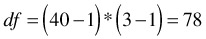The error df is the total df minus the df of each of the factors.

From the ANOVA table, factors A, B, and F are identified as significant. The effects of these factors are shown in Table 9.36 and Figure 9.21.

Table 9.36: The Effect of the Significant Factors

Observed Frequency

% Rate of Occurrence (R.O.)

Cumulative Frequency

Cumulative % R.O.

OK

Good

OK

Good

OK

Good

OK

Good

A1

9

8

3

45

40

15

9

17

20

45

85

100

A2

1

11

8

5

55

40

1

12

20

5

60

100

B1

6

12

2

30

60

10

6

18

20

30

90

100

B2

4

7

9

20

35

45

4

11

20

20

55

100

F1

2

10

8

10

50

40

2

12

20

10

60

100

F2

8

9

3

40

45

15

8

17

20

40

85

100

Total

10

19

11

25

73

100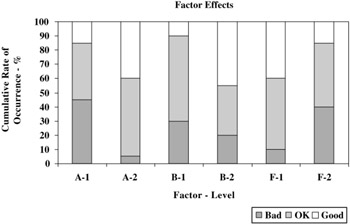Figure 9.21: Factor effects.Although interpretation and use of the ANOVA table in classified attribute analysis is the same as for the non-classified situation, a significant difference does exist in estimating the cumulative rate of occurrence for each class under the optimum condition.

Percentages near 0% or 100% are not additive. The cumulative of occurrence can be transformed using the omega method to obtain values that are additive. In the omega method, the cumulative percentage (p) is transformed to a new value ( ) as follows :

• = -10 log 10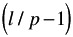[the units of are decibels (db).]

Using this transformation, the estimated cumulative rate of occurrence for each class at the optimum condition (A 2 B 2 F 1 ) is calculated as follows: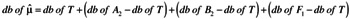The estimated cumulative rate of occurrence for each class for the optimum condition is:

#### Class 1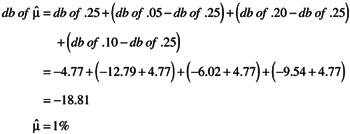#### Class 2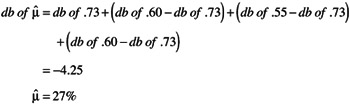These results are summarized in Table 9.37.

Table 9.37: Rate of Occurrence at the Optimum Settings

Class

Cumulative Rate of Occurrence

Rate of Occurrence

1%

1%

OK

27%

26%

Good

100%

73%

### CLASSIFIED VARIABLE ANALYSIS

Classified variable analysis is used when the number of items evaluated is not the same for all test matrix setups. As with classified attribute analysis, the computer analyzes the cumulative frequencies.Four factors (A, B, C and D) are suspected of influencing door closing efforts for a particular car model. An experiment was set up that evaluated each of these factors at three levels. An L9 orthogonal array was used to evaluate the factor levels. Door closing effort ratings were made by a group of typical customers. Each customer was asked to evaluate the doors on a scale of one to three as follows:

Class

Description of Effort

1

Unacceptable

2

Barely acceptable

3

Very good feel

The experimental setup and test results are shown in Table 9.38 and Figure 9.22. The ANOVA analysis for this set of data is shown in Table 9.39.

Table 9.38: Door Closing Effort: Test Setup and Results

A

B

C

D

Number of Ratings

Ratings by Class

Class% Rate of Occurrence

Class Cumulative Frequency (%)

1

2

3

1

2

3

1

2

3

1

1

1

1

5

1

3

1

20

60

20

20

80

100

1

2

2

2

4

2

1

1

50

25

25

50

75

100

1

3

3

3

5

2

3

40

60

40

100

100

2

1

2

3

4

4

100

100

2

2

3

1

4

1

3

25

75

25

100

2

3

1

2

4

1

3

25

75

25

100

3

1

3

2

5

3

2

60

40

60

100

100

3

2

1

3

5

4

1

80

20

80

100

100

3

3

2

1

4

3

1

75

25

75

100

100

Table 9.39: ANOVA Table for Door Closing Effort

Source

df

SS

MS

F Ratio

S'

%

A

4

871.296

217.824

447.277

869.348

48.30

B

4

34.404

8.601

17.661

32.456

1.80

C

4

25.125

6.296

12.928

23.234

1.29

D

4*

4.827

1.207

Error

1782

864.291

0.485

(pooled error)

1786

869.118

0.487

874.962

48.61

Total

1798

1800.000

1.001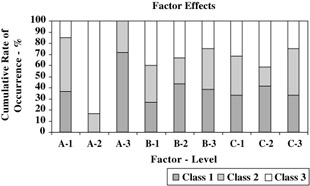Figure 9.22: Factor effects.

From the ANOVA table, factors A, B and C are identified as significant. The effects of these factors are shown in Table 9.40.

Table 9.40: The Effects of the Door Closing Effort

Factor & Level

% Rate of Occurrence

Cumulative % Rate of Occurrence

Class 1

Class 2

Class 3

Class 1

Class 2

Class 3

A1

36.7

48.3

15.0

36.7

85.0

100

A2

16.7

83.3

16.7

100

A3

71.7

28.3

71.7

100.0

100

B1

26.7

33.3

40.0

26.7

60.0

100

B2

43.3

23.3

33.3

43.3

66.6

100

B3

38.3

36.7

25.0

38.3

75.0

100

C1

33.3

35.0

31.7

33.3

68.3

100

C2

41.7

16.7

41.7

41.7

58.4

100

C3

33.3

41.7

25.0

33.3

75.0

100

Total

36.1

31.1

32.8

36.1

67.2

100

The choice of the optimum levels is clear for factors A and B. A 2 and B 1 are the best choices. Two different choices are possible for factor C, depending on the overall goal of the design. If the goal is to minimize the occurrence of unacceptable efforts, C 1 is the best choice. If the goal is to maximize the number of customer ratings of "very good," then C 2 is the best choice. For this example, C 1 will be chosen as the preferred factor setting. The estimated rate of occurrence for each class for the optimum setting, A 2 B 1 C 1 , can be calculated using the omega method. The estimated rates are shown in Table 9.41. The df for the factors are calculated in the same way as with the Classified Attribute Analysis, i.e., df = (the number of levels of that factor - 1) * (the number of classes - 1).

Table 9.41: Rate of Occurrence at the Optimum Settings

Class

Cumulative Rate of Occurrence

Rate of Occurrence

1 (unacceptable)

0%

0%

2 (barely acceptable)

13.4%

13.4%

3 (very good feel)

100%

86.6%

In Classified Variable Analysis, the total number of items evaluated at each condition is not equal. To "normalize" these sample sizes, percentages are analyzed and the "sample size " for each test setup becomes 100 (for 100%). The total df is (the number of "sample sizes" - 1) * (the number of classes - 1). For this example, the total df is: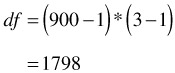The error df is the total df minus the df of each of the factors.### DISCUSSION OF THE DEGREES OF FREEDOM

In both classified attribute analysis and classified variable analysis, the total degrees of freedom are much greater than the number of items evaluated. The interpretation of the F ratios and the calculation of a confidence interval are complicated by the large number of degrees of freedom and will not be addressed here. The analysis techniques for classified responses are not as completely developed as are the techniques for the analysis of continuous data. In Dr. Taguchi's approach, the emphasis is on using the percent contribution to prioritize alternative choices. Although better statistical techniques may be developed to handle classified data, classified attribute and classified variable analyses can be used to identify the large contributors to variation in classified responses.

### MISCELLANEOUS THOUGHTS

As we just mentioned in the discussion of the degrees of freedom, there is no consensus among statisticians regarding the best method to use to analyze classified data. A method that is an alternate to the ones described in this section is to transform the classified data into variable data and analyze the data as described in Section 5. A drawback to this approach is that the relative difference in the transformed values should reflect the relative difference in the classifications, and this is sometimes difficult to achieve. A simple example from the medical field will illustrate this. Four different groups of patients suffering from the same disease are each given a different medicine. The purpose is to determine which medicine is best. The response classes are shown in below:

Class

Description of Effect

A

Patient improves

B

No change in patient

C

Patient dies

If Class A is given a value of 1 and Class B is given a value of 2, what should Class C be given? Is the difference between Classes B and C the same as the difference between Classes A and B? Twice the difference? Three times?

Dr. George Box is of the opinion that this difficulty can be overcome by analyzing the variable data using several different transformations from the classifications. In most instances, the choice of the best response will not be affected by the different relative values placed on the classifications and, in every case, the data will be much easier to analyze and interpret. The example given earlier dealing with classified attribute data will be worked as an example.Three grades are used to evaluate paint appearance of a product. They are "Bad," "OK," and "Good." The classified data are transformed into variable data as follows: Bad = 1; OK = 3; Good = 4. This puts emphasis on avoiding situations that result in "bad" responses. Seven factors (A through G), each at two levels, are evaluated to determine the combination of factor levels that optimizes paint appearance. Five products are evaluated at each testing situation in an L8 orthogonal array. Test results are shown in Table 9.42. The ANOVA analysis for the raw data is shown in Table 9.43. Plotting of the data and inspection of the level averages reveal that the best factor choices are: A 2 B 2 F 1 . The ANOVA analysis for the NTB S/N ratios is shown in Table 9.44.

Table 9.42: OA and Test Setup and Results

Test Setup and Results

A

B

C

D

E

F

G

OK

Good

Transformed Data

1

1

1

1

1

1

1

2

3

1

1

3

3

1

1

1

2

2

2

2

3

2

1

1

1

3

1

2

2

1

1

2

2

4

1

1

1

1

3

1

2

2

2

2

1

1

2

3

3

3

4

4

2

1

2

1

2

1

2

4

1

3

3

3

4

2

1

2

2

1

2

1

1

3

1

1

3

3

4

2

2

1

1

2

2

1

3

2

3

3

3

4

2

2

1

2

1

1

2

1

4

3

4

4

4

Table 9.43: ANOVA for the Raw Data

Source

df

SS

MS

F Ratio

S'

%

A

1

11.03

11.03

14.33

10.26

20.94

B

1

3.03

3.03

3.93

2.26

4.61

C

1*

0.03

0.03

D

1*

2.03

2.03

E

1*

2.03

2.03

F

1

7.23

7.23

9.39

6.46

13.18

G

1*

2.03

2.03

Error

32

21.60

0.68

(pooled error)

36

27.70

0.77

30.01

61.27

Total

39

48.98

1.26

Table 9.44: ANOVA Table for the NTB S/N Ratios

Source

df

SS

MS

F Ratio

S'

%

A

1

23.81

23.81

16.76

22.39

25.18

B

1

23.81

23.81

16.76

22.39

25.18

C

1*

0.39

0.39

D

1*

0.39

0.39

E

1

13.21

13.21

9.29

11.79

13.26

F

1

23.81

23.81

16.76

22.39

25.18

G

1*

3.49

3.49

Error

3

(pooled error)

4.26

1.42

9.95

11.19

Total

7

88.91

12.70

Plotting of the S/N data and inspection of the level averages reveals that the best factor choices are: A 2 B 2 E 2 F 1 . The best choices overall are: A 2 B 2 E 2 F 1 . This compares with the best choice of A 2 B 2 F 1 from the accumulation analysis on page 425.

Each of the methods has one further disadvantage . Using the transformation approach, it is not possible to make a projection of what the distribution of classes would look like at the optimum settings. The accumulation analysis was not able to identify the effect on the standard deviation of the ratings due to factor E. Each approach tells a different part of the story and both should be used to get the full picture.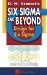Six Sigma and Beyond: Design for Six Sigma, Volume VI
ISBN: 1574443151
EAN: 2147483647
Year: 2003
Pages: 235